# Important Reasoning Ability Questions for IBPS CLERK MAINS EXAM 2017

Dear Banking Aspirant,

The new year has started with positive vibrations and you have to propel your preparations to the next level to ace the IBPS CLERK MAINS Examination which is the initial start for the new born year 2018. So , as you all know we have been providing questions for the IBPS CLERK Mains Examination after the end of the IBPS Clerk Prelims examination, so that the candidates could benefit the aspirants.

Constant preparations is the key to crack the any exams and again as the exams are to be taken seriously as the exams are usually in the highest difficulty range, one has to prepare to the core to crack the exams. Solve these data sufficiency questions which will be helpful in cracking the IBPS CLERK PRELIMS EXAM 2017.

D.1-3) Each of the questions below consist of a statements below it. You have to decide whether the data provided in the statements are sufficient to answer the question and mark the appropriate option as answer.

Q.1) Five persons P, Q, R, S and T are sitting in row 1 facing south. A, B, C, D and E are sitting in row two facing north. A person who is sitting row 1 and the person who are sitting row 2 facing each other. What is the position of E with respect to A?

I. The person who is second to the left of A is facing the person who is immediate right of R.

II. Q sits second to the right of U. Number of persons are in the right of U and right of E are same.

III. Only one person sit between D and B. D and Q are not facing each other.

a) Only I and III are sufficient to answer the question

b) Only II and III are sufficient to answer the question

c) All I, II and III together sufficient to answer the question

d) Only I and II are sufficient to answer the question

e) All I, II and III together not sufficient to answer the question

Q.2) Seven persons A, B, C, D, E, F and G are sitting in a row some of them are facing north and others facing south. Not more than two persons sit together are facing the same direction. What is the position of C with respect to E?

I. B is third to the right of D. F sits second to the right of A. C is not facing south.

II. Only one person sits between B and G. C and G are immediate neighbours. D and G are facing the opposite direction.

III. E sits second to the right of C. Persons who are sitting at the extreme end are facing the opposite direction.

a) Only I and III are sufficient to answer the question

b) Only II and III are sufficient to answer the question

c) All I, II and III together sufficient to answer the question

d) Only I and II are sufficient to answer the question

e) All I, II and III together not sufficient to answer the question

Q.3) Among P, Q, R, S and T, who is third to the right of T?

I. All persons are sitting in a row and facing same direction.

II. All persons are sitting in a circle and facing inside the circle.

III. T is second to the left of Q. S is not an immediate neighbour of P, who is second to the right of Q.

a) None of these

b) Only II and III are sufficient to answer the question

c) Only III and either I or II are sufficient to answer the question

d) Only I and II are sufficient to answer the question

e) All I, II and III together not sufficient to answer the question

D.4-6) Each of the questions below consist of a statements below it. You have to decide whether the data provided in the statements are sufficient to answer the question and mark the appropriate option as answer.

Q.4) Six persons C, D, E, F, G and H are sitting around a circular table. Is all facing inside the circle?

I. Two persons sit between C and G. H is not an immediate neighbour of C who faces inside. E faces the same direction as H.

II. G sits second to the right of E. Immediate neighbours of C faces the same direction. Immediate neighbours of G faces the same direction.

III. Two persons sit between F and H. Immediate neighbours of E faces the same direction.

a) All I, II and III are together not sufficient to answer the question

b) Only II and III are together sufficient to answer the question

c) Only I and III are together sufficient to answer the question

d)Any two of the three statements are sufficient to answer the question

e) All I, II and III together sufficient to answer the question

Q.5) Six persons D, E, F, G, H and I are attending the interview on six different days of the week starting from Sunday. Who is attending the interview on Wednesday?

I. D attends the interview immediately before I. More than one person attends the interview between D and H.

II. Two persons attending the interview between E and F. Number of persons attending the interview before E and after F are equal.

III. More than two persons attending the interview between E and H.

a) Only I and II are sufficient to answer the question

b) Any two of the three statements are sufficient to answer the question

c) Only I and III are sufficient to answer the question

d) Only II and either I or III are sufficient to answer the question

e) All I, II and III are together sufficient to answer the question

Q.6) Seven persons K, L, M, N, O, P and Q are sitting in a row. Some of them are facing north while some of them facing south. How many persons sitting in a row face south?

I. Three persons sit between M and L. N sits second to the left of L and faces south. More than one person sits between M and N. P does not face south. Immediate neighbours of P faces the opposite direction.

II. P sits second to the left of both O and K. M sits at the end of the row. K faces south. Immediate neighbours of L faces the same direction. Persons sit at the end of the row face opposite direction.

III. More than two persons face the same direction.

a) Only I and II are sufficient to answer the question

b) Any two of the three statements are sufficient to answer the question

c) Only I and III are sufficient to answer the question

d) Only III and either I or II are sufficient to answer the question

e) All I, II and III are together sufficient to answer the question

D.7-10) Each of the questions below consist of a statements below it. You have to decide whether the data provided in the statements are sufficient to answer the question and mark the appropriate option as answer.

Q.7) Among A, B, C, D, E and F, how many persons live between A and B (consider they are living in a six storeyed building)?

I. Two persons live between F and C. Neither E nor B lives below C. F lives immediately above E.

II. D lives two floors above E. More than one person lives between B and C.

III. C lives in the odd numbered floors. D lives in the odd numbered floors above F, who does not live below fourth floor.

a) Only I and II are sufficient to answer the question

b) Any two of the three statements are sufficient to answer the question

c) Only I and III are sufficient to answer the question

d) Only I and either II or III are sufficient to answer the question

e) All I, II and III are together sufficient to answer the question

Q.8) Six persons D, E, F, G, H and I are sitting around a circular table. Some of them are facing the centre while some of them are facing away from the centre. Who sits second to the right of G?

I. Two persons sit between F and E. F sits second to the left of I. Immediate neighbours of D face inside. Neither F nor D is an immediate neighbour of I.

II. One person sits between I and H. Immediate neighbours of E face same direction. Immediate neighbours of F face opposite direction of H.

III. D sits second to the right of E. H is an immediate neighbour of E. Immediate neighbours of H face opposite direction. Immediate neighbours of I face opposite direction.

a) Only I and II are sufficient to answer the question

b) Any two of the three statements are sufficient to answer the question

c) Only I and III are sufficient to answer the question

d) Only I and either II or III are sufficient to answer the question

e) All I, II and III are together sufficient to answer the question

Q.9) Students in the class are standing in a row facing the north. How many students are in the class, if total numbers of students in the class do not exceed 200?

I. Samir is twentieth from the left end.

II. Amir is twenty fifth from the right end. Number of persons between Mani and Samir is divisible by 11.

III. Mani is in the middle of Samir and Amir. Number of persons between Amir and Samir is divisible by 7.

a) All I, II and III are together not sufficient to answer the question

b) Only II and III are together sufficient to answer the question

c) Only I and III are together sufficient to answer the question

d)Any two of the three statements are sufficient to answer the question

e) All I, II and III together sufficient to answer the question

Q.10) Nine persons A, B, C, D, E, F, H, I and J are family members. Three married couples are in the family. How is C related to F?

I) E is the brother of F and uncle of A who is the daughter of H who is the uncle of J. D is the mother-in-law of I. F does not have a niece.

II) C is the paternal aunt of I’s husband and sister in law of D. C, D and B are from the first generation. D has two children. C is unmarried.

III) F and H are a

parent of A. F is the sister-in-law of I who is not a father of J.

a) Only I and II are sufficient to answer the question

b) Only I and III are sufficient to answer the question

c) Only I and either II or III is sufficient to answer the question

d) Only II and either I or III is sufficient to answer the question

e) All are required to answer the question

Please check the answers for the above questions from the options given below.

1. (e)

All I, II and III together not sufficient to answer the question.

2. (e)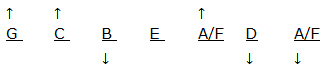3. (c)

From I and III

T        S        Q       R        P

From II and III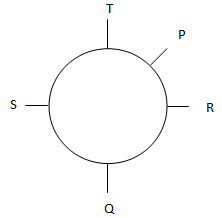4. (a)

From I, II and III

We can’t find the direction of G

5. (e)

From I, II and III

 Sunday G Monday E Tuesday D Wednesday I Thursday F Friday H

6. (a)

From I and II

M (Down)  K(Down)     Q(Up)      P(Up)      L(Down)       O(Up)      N(Down)

7. b

From I and II

 6 B 5 D 4 F 3 E 2 A 1 C

From I and III

 6 A/B 5 D 4 F 3 E 2 A/B 1 C

From II and III

 6 A/B 5 D 4 F 3 E 2 A/B 1 C

8. (d)

From I and II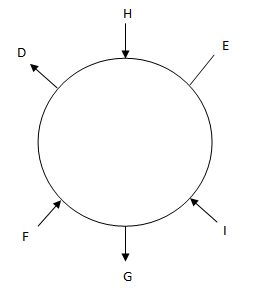From I and III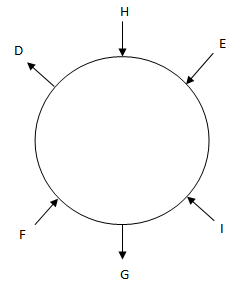9. (e)

From all I, II and III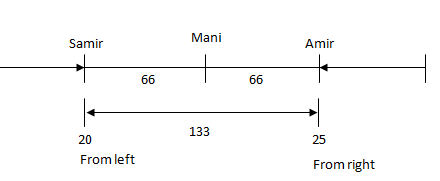20 + 133 + 25 = 178x

Q.10) (a)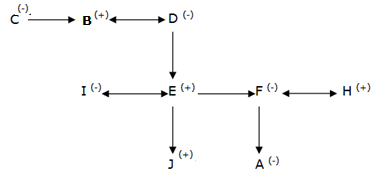I & II

Other Important Question set regarding Reasoning Ability :

Practice Important Reasoning Ability Questions For IBPS SO Prelims Exam 2017

Practice Important Reasoning Ability Questions For IBPS SO Prelims Exam 2017

Practice Important Reasoning Ability Questions For IBPS SO Prelims Exam 2017